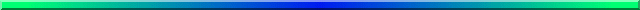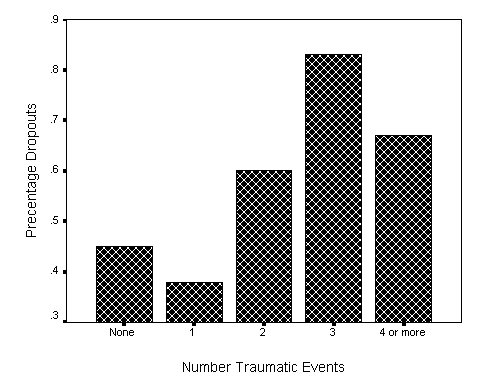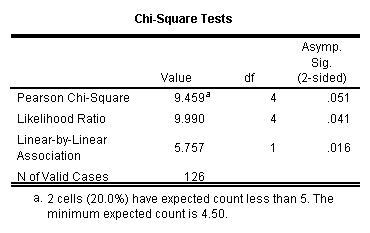# Ordinal Chi-Square

## David C. HowellChi-square is an important statistic for the analysis of categorical data, but it can sometimes fall short of what we need. If you apply chi-square to a contingency table, and then rearrange one or more rows or columns and calculate chi-square again, you will arrive at exactly the same answer. That is as it should be, because chi-square is does not take the ordering of the rows or columns into account.

But what do you do if the order of the rows and/or columns does make a difference? How can you take that ordinal information and make it part of your analysis? An interesting example of just such a situation was provided in a query that I received from Jennifer Mahon at the University of Leicester, in England.

Ms Mahon collected data on the treatment for eating disorders. She was interested in how likely participants were to remain in treatment or drop out, and she wanted to examine this with respect to the number of traumatic events they had experienced in childhood. Her general hypothesis was that participants who had experienced more traumatic events during childhood would be more likely to drop out of treatment.

The data from this study are shown below. I have taken the liberty of altering them slightly so that I don't have to deal with the problem of small expected frequencies at the same time that I am trying to show how to make use of the ordinal nature of the data. The altered data are still a faithful representation of the effects that she found.

 Number of  Traumatic Events 0 1 2 3 4+ Total Dropout 25 13 9 10 6 63 Remain 31 21 6 2 3 63 Total 56 34 15 12 9 126

At first glance we might be tempted to apply a standard Pearson's chi-square test to these data, testing the null hypothesis that dropping out of treatment is independent of the number of traumatic events the person experienced during childhood. If we do that we find a chi-square of 9.459 on 4 df, which has an associated probability of .051. Strictly speaking, this result does not allow us to reject the null hypothesis, and we might conclude that traumatic events are not associated with dropping out of treatment. However, that answer is a bit too simplistic.

Notice that Trauma represents an ordered variable. Three traumatic events are more than 2, 2 traumatic events are more than 1, and so on. If we look at the percentage of participants who dropped out of treatment, as a function of the number of traumatic events they had experienced as children, we have the following plot.This plot shows that dropouts appear to increase with increasing number of traumatic events. But this trend was not allowed to play any role in our calculated chi-square. What we want is a statistic that does take order into account.

## A Correlational Approach

There are several ways we can accomplish what we want, but they all come down to assigning some kind of ordered metric to our independent variables. Dropout is not a problem because it is a dichotomy. We could code dropout as 1 and non-dropout as 2, or dropout as 1 and non-dropout as 0, or any other two values we like. The result will not be affected by our choice of values. When it comes to the number of traumatic events, we could simply use the numbers 0, 1, 2, 3, and 4. Alternatively, if we thought that 3 or 4 traumatic events would be much more important than 1 or 2, we might use 0, 1, 2, 4, 6. In practice, as long as we chose numbers that are monotonically increasing, and are not very extreme, the result will not change much as a function of our choice. I will choose to use 0, 1, 2, 3, and 4.

Now that we have established a metric for each independent variable, there are several different ways that we could go. We'll start with one that has good intuitive appeal. We will simply correlate our two variables. Each participant will have a score of 0 or 1 on Dropout, and a score between 0 and 4 on Trauma. The Pearson correlation between those two measures is -.215, which has an associated probability under the null of .016. This correlation is significant, and we can reject the null hypothesis of independence.

As a slight digression, if you are unhappy with the idea of specifying a particular metric for Trauma, although you do agree that it is an ordered variable, you could calculate Kendall's tau instead of Pearson's r. Tau would be the same for any set of values you assign to the levels of Trauma, assuming that they increased across the levels of that variable. For our data tau would be -.169, with a probability of .04. So the relationship would still be significant even if we are only confident about the order.

Agresti (1996) presents the approach that we have just adopted and shows that

M2 = (N - 1)r2 ,

where M2 is a chi-square statistic on 1 degree of freedom, r is the correlation between Dropout and Trauma, and N is the sample size.. For our example this becomes

M2 = c2(1)  = 125*(-.215)2 = 5.757

which has an associated probability under the null of .016.

We can go one step further before leaving this approach. We know that the overall Pearson chi-square on 4 df = 9.459. We also know that we have just calculated a chi-square = 5.757 on 1 df associated with the linear relationship between the two variables. That linear relationship is part of the total chi-square, and if we subtract the linear component from the overall chi-square we obtain

 df Chi-square Pearson 4 9.459 Linear 1 5.757 Deviation from linear 3 3.702

The departure for linearity is itself a chi-square = 3.702 on 3 df, which has a probability under the null of .295. Thus we do not have any evidence that there is anything other than a linear trend underlying these data. (In other words, the relationship between Trauma and Dropout is not curvilinear.)

Agresti (1996) has an excellent discussion of the approach taken here, and he makes the interesting point that for small to medium sample sizes, the standard Pearson chi-square is more sensitive to small sample size than is the ordinal chi-square that we calculated. In other words, although some of the cells in the contingency table are small, I am more confident of the ordinal (linear) chi-square = 5.757 than I can be of the Pearson chi-square of 9.459.

You can calculate the chi-square for linearity using SPSS. If you request the chi-square statistic from the statistics dialog box, your output will include the Pearson chi-square, the Likelihood Ratio chi-sq, and Linear-by-Linear Association. The SPSS printout of the results for the data from Mahon is shown below. You will see that the Linear-by-Linear Association measure = 5.757, which is the same as the chi-square that we calculated using (N - 1)r2 .### Alternative Approaches

There are a number of other ways to approach the problem of ordinal variables in a contingency table. In some cases only one of the variables is ordinal and the other is nominal. (Remember that dichotomous variables can always be treated as ordinal without affecting the analysis.) In other cases one of the variables is clearly an independent variable while the other is a dependent variable.  An excellent discussion of some of these methods can be found in Agresti, 1996.

Many articles  in the literature refer to a paper by Maxwell (1961) as a source for dealing with ordinal data. With one minor exception, Maxwell's approach is the one advocated here, though it is difficult to tell from his description because his formulae were selected for computational ease.

Agresti, A. (1996) An introduction to categorical data analysis. New York: Wiley.

Maxwell, A. R. (1961) Analysing qualitative data. London: Metheun & Co.Send mail to: David.Howell@uvm.edu)

Last revised 6/28/2015